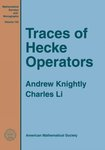## Faculty and Staff Monograph Publications#### Title

Traces of Hecke Operators

#### Description

The Fourier coefficients of modular forms are of widespread interest as an important source of arithmetic information. In many cases, these coefficients can be recovered from explicit knowledge of the traces of Hecke operators. The original trace formula for Hecke operators was given by Selberg in 1956. Many improvements were made in subsequent years, notably by Eichler and Hijikata. This book provides a comprehensive modern treatment of the Eichler-Selberg/Hijikata trace formula for the traces of Hecke operators on spaces of holomorphic cusp forms of weight $\mathtt{k}>2$ for congruence subgroups of $\operatorname{SL}_2(\mathbf{Z})$. The first half of the text brings together the background from number theory and representation theory required for the computation. This includes detailed discussions of modular forms, Hecke operators, adeles and ideles, structure theory for $\operatorname{GL}_2(\mathbf{A})$, strong approximation, integration on locally compact groups, the Poisson summation formula, adelic zeta functions, basic representation theory for locally compact groups, the unitary representations of $\operatorname{GL}_2(\mathbf{R})$, and the connection between classical cusp forms and their adelic counterparts on $\operatorname{GL}_2(\mathbf{A})$. The second half begins with a full development of the geometric side of the Arthur-Selberg trace formula for the group $\operatorname{GL}_2(\mathbf{A})$. This leads to an expression for the trace of a Hecke operator, which is then computed explicitly. The exposition is virtually self-contained, with complete references for the occasional use of auxiliary results. The book concludes with several applications of the final formula.

9780821837399

2006

#### Publisher

American Mathematical Society

Providence, RI

#### Keywords

Hecke operators, Trace formulas, Number theory

#### Disciplines

Mathematics | Number Theory